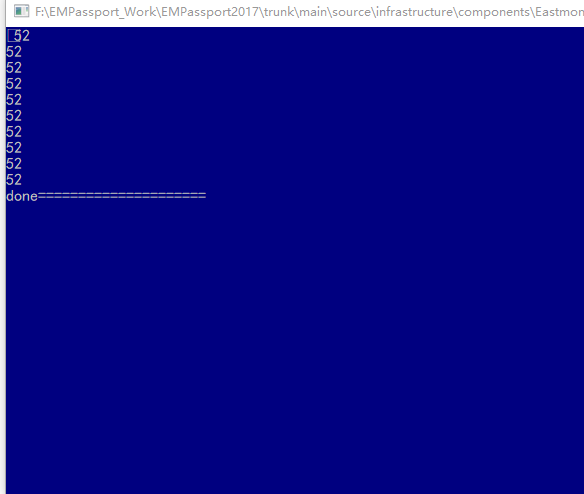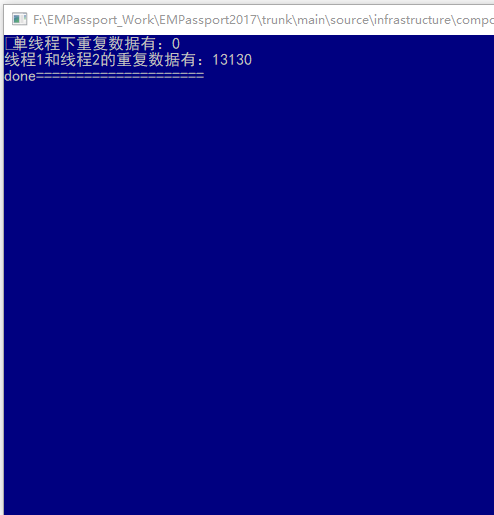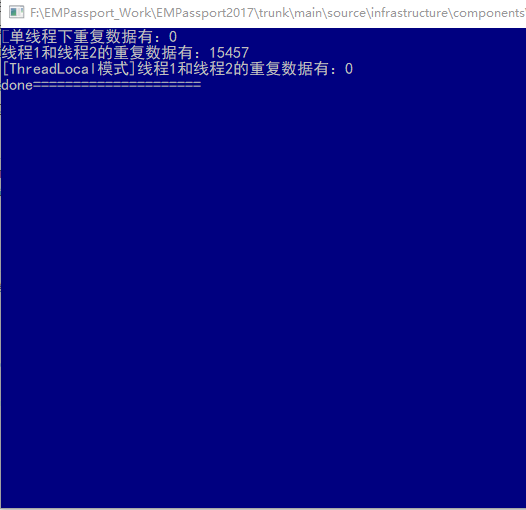# C# Random类的正确应用

## Random类介绍

Random类一个用于产生伪随机数字的类。这里的伪随机表示有随机性但是可以基于算法模拟出随机规律。
Random类的构造方式有两种。

• Random r= new Random()。会以当前系统时间作为默认种子构建一个随机序列
• Random r = new Random(unchecked((int)DateTime.Now.Ticks));。自定义一个种子，通常会使用时间Ticks。

## 随机性保证

    /// <summary>
/// 错误的Random构建。
/// </summary>
{
//正确做法应当将 Random构建防止循环外。
//Random创建间隔时间极短的情况下，随机算法序列会基本一致，倒是随机性也是一致的
//var r = new Random();
for (int i = 0; i < 10; i++)
{
var r = new Random();
var val = r.Next(1, 100);
Console.WriteLine(val);
}
}Instead of instantiating individual Random objects, we recommend that you create a single Random instance to generate all the random numbers needed by your app.


    /// <summary>
/// 生成一个10位随机数
/// 设定了一定的复杂性，保证单线程下随机数不重复
/// </summary>
/// <param name="random">Random.</param>
/// <returns>随机数.</returns>
private static string GenerateRandomStr(Random random)
{
string source = "ABCDEFGHIKLMNOPQRTUVWXYZabcdefghiklmnopqrtuvwxyz";
int length = 10;
var list = Enumerable.Repeat(source, length)
.Select(s => s[random.Next(s.Length)]).ToArray();
return new string(list);
}
/// <summary>
/// 单线程基本可以保证唯一性
/// </summary>
{
//正确做法应当将 Random构建防止循环外。
//Random创建间隔时间极短的情况下，随机算法序列会基本一致，倒是随机性也是一致的
var r = new Random();
ConcurrentBag<string> list = new ConcurrentBag<string>();
for (int i = 0; i < 20000; i++)
{
var val = GenerateRandomStr(r);
}

Console.WriteLine($"单线程下重复数据有：{20000 - list.Distinct().Count()}"); } /// <summary> /// 多线程下的Random构建。 /// Bad案例，Random非线程安全 /// 多线程高并发情况下，会出现概率重复 /// </summary> public static void Bad_Random_In_MultThreads() { var r = new Random(unchecked((int)DateTime.Now.Ticks)); ConcurrentBag<string> list = new ConcurrentBag<string>(); var t1 = Task.Run(() => { for (int i = 0; i < 10000; i++) { var val = GenerateRandomStr(r); list.Add(val); } }); var t2 = Task.Run(() => { for (int i = 0; i < 10000; i++) { var val = GenerateRandomStr(r); list.Add(val); } }); Task.WaitAll(t1, t2); Console.WriteLine($"线程1和线程2的重复数据有：{20000 - list.Distinct().Count()}");
}//利用ThreadLocal 实现每个线程下Random独有
//再通过seed原子性变更，保证每个Random的seed不同而生成的随机数列也不同
private static int seed = 100;

/// <summary>
/// 多线程下的Random构建。
/// </summary>
{
ConcurrentBag<string> list = new ConcurrentBag<string>();

{
for (int i = 0; i < 10000; i++)
{
}
});

{
for (int i = 0; i < 10000; i++)
{
}
});

}posted on 2020-11-25 10:50  gt1987  阅读(949)  评论(2编辑  收藏  举报